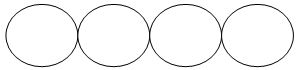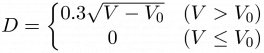## Day 1, Problem 1 - Ceramic Necklace

Ancient tribes heated up a rare type of clay to produce ceramic disks with identical diameters. These disks are strung together to make necklaces by being joined along their diameters with no spaces or overlaps between them. Each necklace is made up of at least one disk.

The figure below depicts four rings of the same size, linked together to form a necklace with a length four times that of a single disk's diameter.Each heat-crafted ceramic disk has a fixed thickness, while the diameter D and the volume V are related by the following formula:$\displaystyle D=\left\{\begin{matrix}0.3\sqrt{V-V_0}&(V>V_0)\\0&(V\leq V_0)\end{matrix}\right.$

where V0 is the volume of clay lost when heating each disk, in the same units as V. If the amount of material is less than or equal to V0, then the disk cannot be produced.

Ex: Let Vtotal = 10, V0 = 1. If only one disk is produced, then V = Vtotal = 10, and D = 0.9. If the clay is equally split up into two portions, then the volume of each portion V = Vtotal / 2 = 5, the diameter of each disk D' = 0.3√5 − 1 = 0.6, and the total length of the necklace will be 1.2.

Given the total volume of clay and the amount lost for a single disk, and given that the number of disks produced is different, as well that the lengths of the final necklaces are also different, please calculate the number of disks produced that would maximize the length of the necklace.

### Input Format

The input consists of two lines, each containing a single integer. The first line contains Vtotal, the total volume of clay (0 < Vtotal < 60000). The second line contains V0, the volume of clay lost for producing each disk (0 < V0 < 600).

### Output Format

The output should consist of one integer - the number of disks in the necklace with the maximally obtainable length. If it is not possible to produce disks or the answer is not unique (i.e. there exists two or more ways to obtain the longest possible necklace), output the number 0.

### Sample Input 1

10
1


### Sample Output 1

5


### Sample Input 2

10
2


### Sample Output 2

0


Point Value: 5
Time Limit: 1.00s
Memory Limit: 16M